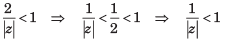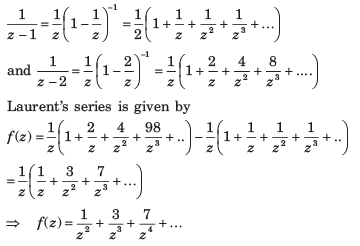Courses

# Complex Variables - MCQ Test

## 15 Questions MCQ Test RRB JE for Electronics & Communication Engineering | Complex Variables - MCQ Test

Description
This mock test of Complex Variables - MCQ Test for Railways helps you for every Railways entrance exam. This contains 15 Multiple Choice Questions for Railways Complex Variables - MCQ Test (mcq) to study with solutions a complete question bank. The solved questions answers in this Complex Variables - MCQ Test quiz give you a good mix of easy questions and tough questions. Railways students definitely take this Complex Variables - MCQ Test exercise for a better result in the exam. You can find other Complex Variables - MCQ Test extra questions, long questions & short questions for Railways on EduRev as well by searching above.
QUESTION: 1

### The integration of f (z) = x2 + ixy from A(1, 1) to B(2, 4) along the straight line AB joining the two points is

Solution:

x = at + b, y = ct + d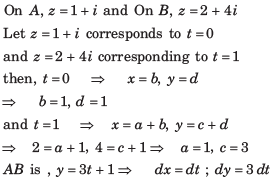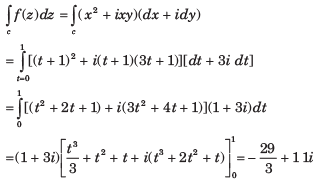QUESTION: 2

###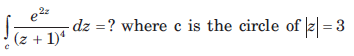​ ​ ​

Solution:

We know by the derivative of an analytic function that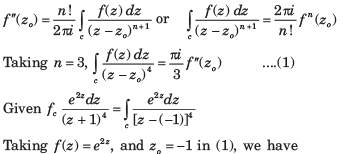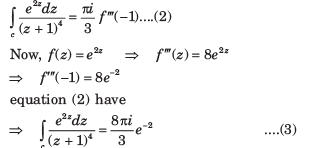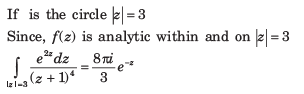QUESTION: 3

###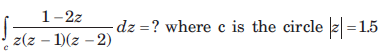Solution: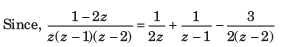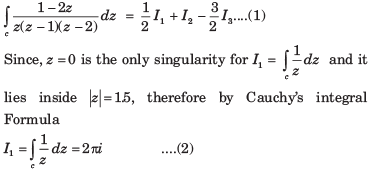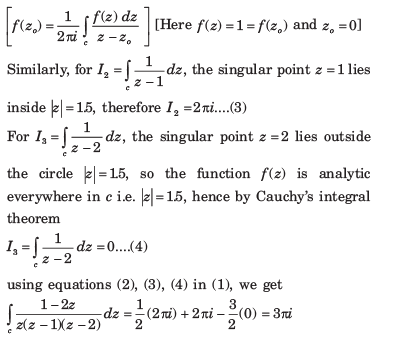QUESTION: 4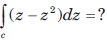where c is the upper half of the circle z = 1

Solution:

Given contour c is the circle |z| = 1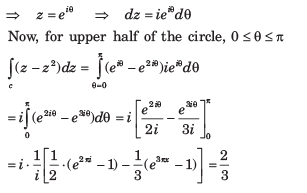QUESTION: 5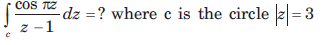Solution:

Let f (z) = cosπz then f(z) is analytic within and on |z| =3, now by Cauchy’s integral formula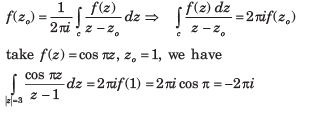QUESTION: 6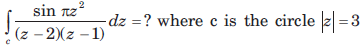Solution: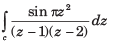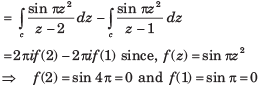QUESTION: 7

The value of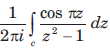around a rectangle with vertices at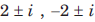is

Solution: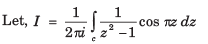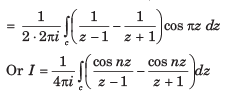QUESTION: 8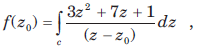where c is the circle x2 + y2 = 4

Que: The value of f(3) is

Solution: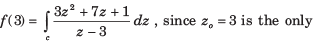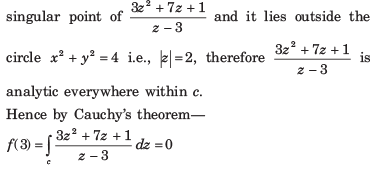QUESTION: 9where c is the circle x2 + y2 = 4

Que: The value of f' (1 - i) is

Solution:

The point (1 - i) lies within circle |z| = 2 ( ... the distance of 1 - i i.e., (1, 1) from the origin is √2 which is less than 2, the radius of the circle).

Let Ø(z) = 3z2 + 7z + 1 then by Cauchy’s integral formula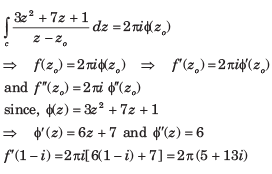QUESTION: 10

Expand the given function in Taylor’s series.

Que: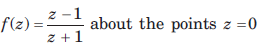Solution: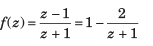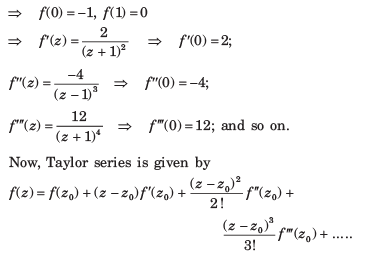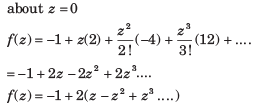QUESTION: 11

Expand the given function in Taylor’s series

Que: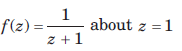Solution: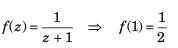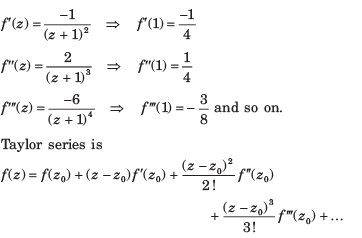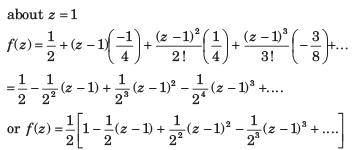QUESTION: 12

Expand the given function in Taylor’s series.

Que: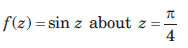Solution: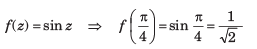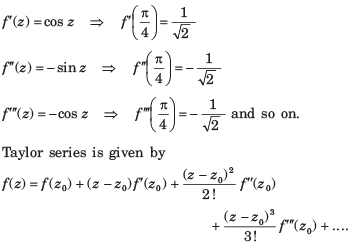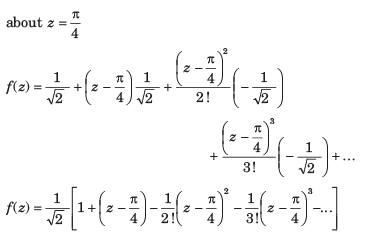QUESTION: 13

If |z + 1|  < 1, then z-2 is equal to

Solution: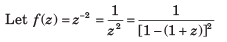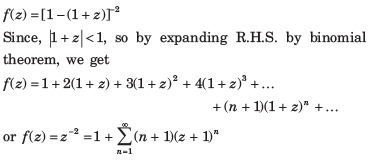QUESTION: 14

Expand the function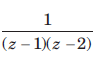in Laurent’s series for the condition given in question.

Que: 1 < |z| < 2

Solution: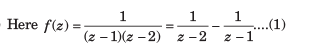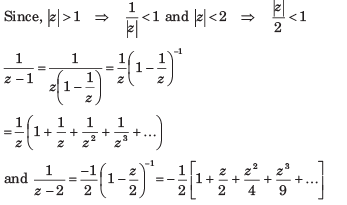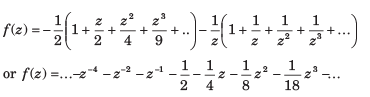QUESTION: 15

Expand the functionin Laurent’s series for the condition given in question.

Que: |z| > 2

Solution: